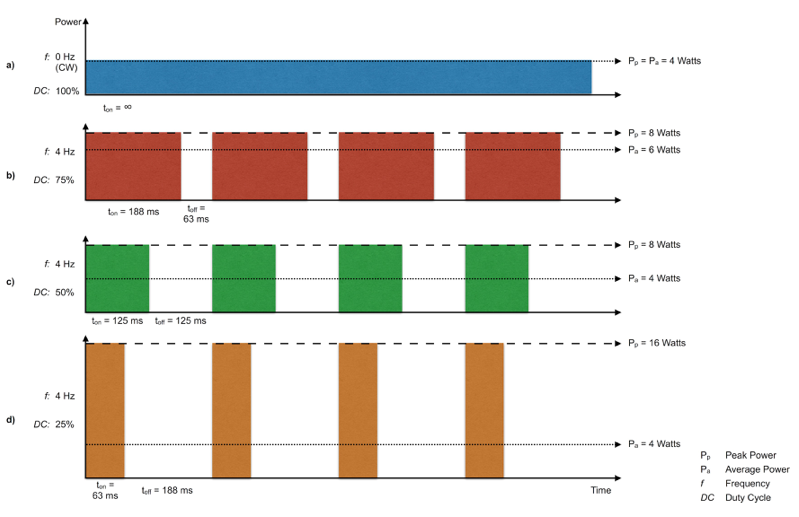• Pulse width ( $t_{on}$ ) and dark period ( $t_{off}$ ) combine to yield more common paramteres - Frequency and Duty Cycle - which, combined with peak power ( $P_{p}$ ), can be used to calculate average power ( $P_{a}$ ).
• $DC = \frac {t_{on}}{t_{on} + t_{off}} = \frac{P_a}{P_p}$